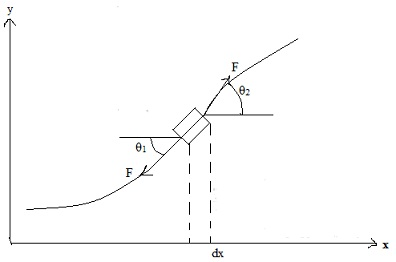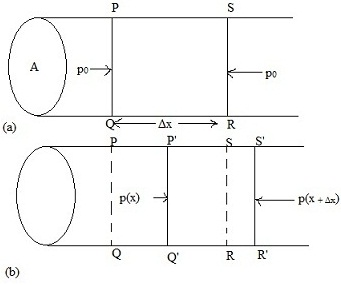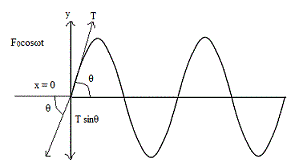#### One dimensional progressive waves, Physics tutorial

Waves on a Stretched String:

Consider the uniform string, having mass per unit length m, stretched by the force F. Let us select x -axis along length of string in its equilibrium state. Assume that string is plucked so that part of it is normal to length of the string, i.e. along y -axis. When string is released it results in wave motion. To know speed of this wave expect that interplay of inertia and elasticity of medium will find out wave speed. For the stretched string, elasticity is estimated by the tension in string and inertia is estimated by m.Using dimensional analysis, illustrate that

v = K√F/m

Where K is some dimensionless constant. Assume that string is distorted slightly so that magnitude of tension on string fundamentally remains unchanged. Direction of (tension) force differs along element under consideration. This is due to string is curved. This signifies that tension forces pulling at opposite ends of element, though of the same magnitude, don't exactly cancel out. To compute net force along x and y-axis, we resolve F in rectangular components. Difference in x and y components of tension between right and left ends of element is respectively provided by

Fx = Fcosθ2 - Fcosθ1 and Fy = Fsinθ2 - Fsinθ1

Where θ1 and θ2 are angles which tangents drawn at the ends make with horizontal. For small oscillations (θ ≤ 40). cosθ1 ≈ cosθ2 ≈ 1

This signifies that there is no net force in x -direction, Fx = 0 and string will be very almost horizontal. This signifies that sines of angles are very almost same as their tangents, i.e.

sinθ1 ≈ tanθ1

sinθ2 ≈ tanθ2

But tangents of two angles are just slopes of the string - or derivatives dy/dt at the ends of element under consideration. Then, -component of the force on element is about

Fy = F(tanθ2 - tanθ1)

F[((dy(x,t)/dx)|x+Δx) + (dy(x,t)/dx)|x]

The quantity in parentheses is just change in first derivative from one end of the interval Δx to other. Dividing that change by Δx provides, in limit Δx→0, the rate of change of the first derivative.

We call a derivative taken with respect to one variable while others are remained constant a partial derivative. Denote partial derivatives with the symbol ∂ in place of usual symbol d. Then, Equation can be rewritten as:

Fy = F(∂2y(x,t)/∂x2)Δx

This equation provides net force on segment Δx. By Newton's second law of motion, we can compare this force to product of mass and acceleration of segment. Mass of the segment of length Δx is mΔx . Then, we can write:

mΔx (∂2y(x,t)/∂t2) = F(∂2y(x,t)/∂t2)Δx

Cancelling Δx on both sides, we get

(∂2y(x,t)/∂x2) = (m/F)(∂2y(x,t)/∂t2)

F/m has dimensions of velocity square.

Let us suppose that harmonic wave explained by moves on string.

y(x,t) = asin(ω0t - kx)

Compute second partial derivatives of particle displacement:

2y/∂x2 = -k2asin(ω0t - kx) and ∂2y/∂t2 = -ω02asin(ω0t - kx) on simplification

k2 = m/Fω02

0/k)2 = F/m

v = ω0/k = √F/m

This relation states that velocity of (transverse) wave on the stretched string depends on tension and mass per unit length of string, not on wavelength or time period.

(∂2y(x,t)/∂x2 = (1/v2)(∂2y/∂t2)

This equation states one dimensional wave equation.

Waves in a Fluid:

Let us consider a fixed mass of a fluid of density ρ contained in a long tube of cross sectional area A and under pressure p0. As for a string, we shall consider a small element (column) of the fluid. Let us assume that the column is at rest and is contained in the region PQRSP extending between planes at x and x + Δx; then mass of the column PQRSP is ΔρxA. You can do so by placing a vibrating tuning fork at its one end or displacing the fluid to the right using a piston. As the wave passes through the column under consideration, its pressure, density and volume change.Let us suppose that in time t planes PQ and SR move to P'Q' and S'R', respectively, as shown in figure. If planes PQ and SR are displaced through ψ(x) and ψ(x + Δx) change in thickness, Δl, is

Δl = ψ(x + Δx) - ψ(x) = ∂ψ/∂xΔx

In writing this expression we have utilized Taylor series expansion for ψ(x + Δx) about ψ(x). This signifies that change in volume ΔV is

ΔV = AΔl = AΔx∂ψ/∂x

Volume strain that is stated as change in volume per unit volume, is provided by:

ΔV/V = -(AΔ∂ψ/∂x)/(AΔx) = -∂ψ/∂x

This occurs as the pressure on its two sides doesn't balance. Let difference of pressures be p(x + Δx) - p(x).

Net force acting on column is, thus A[p(x + Δx) - p(x)]. Using Taylor series and retaining only first order term in Δx, we can write

F = A(∂p(x)/∂x)Δx

= A∂(Δp)/∂x)Δx

Where p0 is equilibrium pressure and Δp is excess pressure because of the wave.

ρΔxA∂2ψ/∂t2 = AΔx(∂(Δp)/∂x)

To states this result in the familiar form, we note that Δp and E, bulk modulus of elasticity, are joined by relation

E = stress/volume strain = -Δp/(ΔV/V)

Negative sign is included to account for fact that when pressure increases, volume decreases. This makes sure that E is positive. We can rewrite it as

Δp = -E(ΔV/V)

On substituting for ΔV/V we get:

Δp = -E∂ψ/∂x

2ψ/∂t2 = (E/ρ)(∂2ψ(x,t)/∂x2

If we identify v = √E/ρ as speed of longitudinal waves. Wave speed is determined only by F and ρ; two properties of medium through which wave is propagating.

a) Sound Waves in a Gas:

For the gaseous medium, like air, volume elasticity depends on thermodynamic changes arising in medium, when the longitudinal wave is propagation. These changes can be isothermal or adiabatic. For sound waves, Newton supposed that changes in medium are isothermal. For the isothermal change, you can check using Boyle's law that volume elasticity equals equilibrium pressure. Then, we can write

v = √p0

This is called as Newton's formula for velocity of sound.

For adiabatic change, E is γ times pressure, where γ is ratio of specific heats at constant pressure and at constant volume, i.e. E =γp0. Then Equation becomes:

v = √γp0

Why heat doesn't have time to flow from the compression to a rarefaction and equalize temperature everywhere. To get this condition, heat has to flow a distance of one-half wavelength in time much shorter than one-half of period of oscillation. This means that we would need

vheat>>vsound

As heat flow is mostly because of conduction, speed of air molecules is provided by

vrms = √kHT/M

Where M is mass of air molecules and T is absolute temperature. We can similarly write

vsound = √γkHT/M

Therefore, even if air molecules travel a distance of λ/2, they won't be able to transfer heat in time. In practice they move arbitrarily in zig-zag paths of order of 10-5cm and as long as λ > 10-5cm, adiabatic flow is a very good approximation. Shortest wavelength for audible sound (1.6cm) corresponds to 20 kHz.

b) Waves in a Uniform Rod:

For the solid elastic rod, changes occur only in length; volume remaining almost constant. Bulk modulus is replaced by Young's modulus stated as

Y = stress/longitudinal strain = Δp/(Δl/l)

Equation modifies to

2ψ/∂t2 = (Y/ρ)(∂2ψ/∂x2)

This is equation of wave motion in rod. Velocity of longitudinal waves is provided

v = √Y/ρ

This illustrates that v is independent of cross-sectional area of rod.

Wave motion and impedance:

When wave travels through a medium, the medium opposes its motion. This resistance to wave motion is known as wave impedance. Impedance offered to transverse waves travelling on strings is known as Characteristic Impedance. Generally, impedance offered to longitudinal (sound) waves in air is known as acoustic impedance. Moreover, each particle in motion tries to make succeeding particle vibrate by transferring energy. Similarly, each particle at rest tends to slow down neighboring particle. That is, a vibrating particle experiences the dragging force that is similar to viscous force. According to Newton's third law of motion, it will be equal to driving force F. When oscillations of particles are small, model viscous force on basis of stokes' law and write

F = Zv

Constant of proportionality Z is called the wave impedance. From this equation it is clear that impedance is numerically equal to the driving force which imparts unit velocity to a particle. We will now consider some specific examples.

Impedance Offered by Strings: Transverse Waves:

Let us consider the wave travelling on the stretched string. Let us select x -axis along length of string. Transverse waves are generated by applying the harmonic force F = F0cosωot at end x = 0 of string. Displacement of particles of string at position x and at time t is given by EqLet T be tension in the string. Vertical component of tension (T) along the negative y direction is equal to applied transverse force (to give zero resultant force at the x = 0 end of string):

F0cosωt = -Tsinθ

For small values of θ (< 50), sinθ ≈ tanθ, so that we can write

F0cosω0t = -Ttanθ

Tangent (or slope) is stated at x = 0 end of the string. We can associate (dy/dx) to (dy/dt) :

dy/dx = -(k/ω0)(dy/dt):

After simplifying we get

F0cosω0t = (Tk/ω0)aω0cosω0t

Writing aω0 = v0 as velocity amplitude of wave, above equation reduces to

F0cosω0t = Tv0/vcosω0t

Giving

F0 = Tv0

F0/vo = T/v

This result states ratio of amplitude of applied force to the amplitude of particle velocity for transverse waves in terms of tension in string and particle velocity. This result can be utilized to get the expression for characteristic impedance (Z) of string which is stated as:

Z = amplitude of the transverse applied force(F0)/ transverse velocity amplitude of the wave(v0)

Z = F0/v0 = T/v

This result illustrates that characteristic impedance has units of Nm s-1 but its dimensions are MT-1

As v = √T/m where m is mass per unit length of the string.

Z = T/v = √Tm

Alternatively, if we eliminate T we can write

Z = v2m/v = mv

Characteristic impedance is run by mass per unit length of string and the tension in it. This signifies that a sonometer wire will present different impedance when it is loaded by different weights.

Impedance Offered by Gases: Sound Waves:

For sound waves propagating in a gas, the role played by excess pressure due to the wave is analogous to that of applied force in case of a transverse wave. So we define the acoustic impedance as:

Z = excess pressure due to a sound wave/particle velocity

(Δp)/(∂ψ/∂t)

It means that dimensionally Z is the ratio of force per unit area to velocity.

The excess pressure Δp experienced by the medium when a longitudinal wave propagates through it is given by

Δp = -E((∂ψ/∂x)

where E is the Bulk modulus of elasticity of the medium. This means that to know Z, we must compute ∂ψ/∂t and ∂ψ/∂x. To do so, we recall that the particle displacement for a longitudinal wave travelling in the +ve x -direction is written as

ψ(x,t) = asin[2π/λ(vt - x)]

Differentiating it with respect to x and t, we get

∂ψ/∂x = -a2π/λcos[2π/λ(vt - x)]

∂ψ/∂t = a(2πv/λ)cos[2π/λ(vt - x)]

Δp = Ea(2π/λ)cos[2π/λ(vt - x)]

On substituting for Δp and ∂ψ/∂t from we find that the acoustic impedance is given by

Z = (Δp)/(∂ψ/∂t) = (Ea(2π/λ)cos[(2π/λ)(vt - x)])/(a(2πv/λ)cos[2π/λ(vt - x)])

= E/v

Where v is the wave velocity. This result illustrates that units of acoustic impedance are Nm-3s and dimensions are ML-2T-1 the wave velocity is provided by:

v = √E/ρ

Where ρ is density of the medium. Therefore acoustic impedance Z can also be stated as:

Z = E/v = √E/ρ = ρv

Waves in two and three dimensions:

The waves are constrained to move along the string whereas particles vibrate in perpendicular direction. But all musical instruments are not stringed. Particles of membrane vibrate along direction of applied force. But tension in membrane creates the disturbance to spread over surface. That is, waves on stretched membranes ate two dimensional (2-D). Likewise, surface waves or ripples on water, caused by dropping the pebble into the quite pond, are 2-D. In such cases, displacement is the function of x, y and t, i.e. ψ = ψ(x, y, t). As forces along x and y axes act independently, each one will contribute analogous term to wave equation so that modifies to ∂2ψ(x, y, t)/∂t2 = F/ρ(∂2/∂x2 + ∂2/∂y2)ψ(x, y, t).

Solution of this equation is

ψ(x, y, t) = asin(ω0t - k.r)

Where k.r = (kxi + kyj). (xi + yj) = kxx + kyy

Tutorsglobe: A way to secure high grade in your curriculum (Online Tutoring)

Expand your confidence, grow study skills and improve your grades.

Since 2009, Tutorsglobe has proactively helped millions of students to get better grades in school, college or university and score well in competitive tests with live, one-on-one online tutoring.

Using an advanced developed tutoring system providing little or no wait time, the students are connected on-demand with a tutor at www.tutorsglobe.com. Students work one-on-one, in real-time with a tutor, communicating and studying using a virtual whiteboard technology.  Scientific and mathematical notation, symbols, geometric figures, graphing and freehand drawing can be rendered quickly and easily in the advanced whiteboard.

Free to know our price and packages for online physics tutoring. Chat with us or submit request at info@tutorsglobe.com